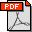digilib@itb.ac.id +62 812 2508 88002020 TA PP CALVIN ORLIANDO 1
Terbatas  Suharsiyah
» Gedung UPT PerpustakaanABSTRAK Calvin Orliando
Terbatas  Suharsiyah
» Gedung UPT Perpustakaan

This study discusses the determination of stabilized flow coefficient (C) where C also stated in the Rawlins and Schellhardt equation which tested in the reservoir model that has a low porosity and low permeability model that is usually found in Unconventional Reservoir. In determining the flow coefficient this time, a deliverability test method which proposed by Hashem and Kazemi was used during the transient period of a gas well. In addition, in determining the deliverability exponent (n) used the least squared analysis equation derived by Johnston and Lee in the determination of C stabilized so that from each value of n there will be supporting data for determining stabilized flow coefficient. Finally, from application and method previous will determine the flow coefficient value based on reservoir model time stabilization which will later be compared with the John Lee equation and IPR constructs from the model and from John Lee.